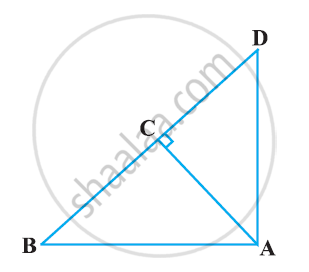Share

# In Figure, ABD is a triangle right angled at A and AC ⊥ BD. Show that AD^2 = BD × CD - CBSE Class 10 - Mathematics

#### Question

In Figure, ABD is a triangle right angled at A and AC ⊥ BD. Show that AD2 = BD × CD#### Solution

In ΔDCA and ΔDAB, we have

∠DCA = ∠DAB (Each equals to 90°)

∴ ΔDCA ~ ΔDAB [By AA similarity criterion]

⇒ (DC)/(DA) = (DA)/(DA)

⇒ AD2 = BD × CD

Is there an error in this question or solution?

#### APPEARS IN

NCERT Solution for Mathematics Textbook for Class 10 (2019 to Current)
Chapter 6: Triangles
Ex. 6.50 | Q: 3.3 | Page no. 150

#### Video TutorialsVIEW ALL 

Solution In Figure, ABD is a triangle right angled at A and AC ⊥ BD. Show that AD^2 = BD × CD Concept: Pythagoras Theorem.
S